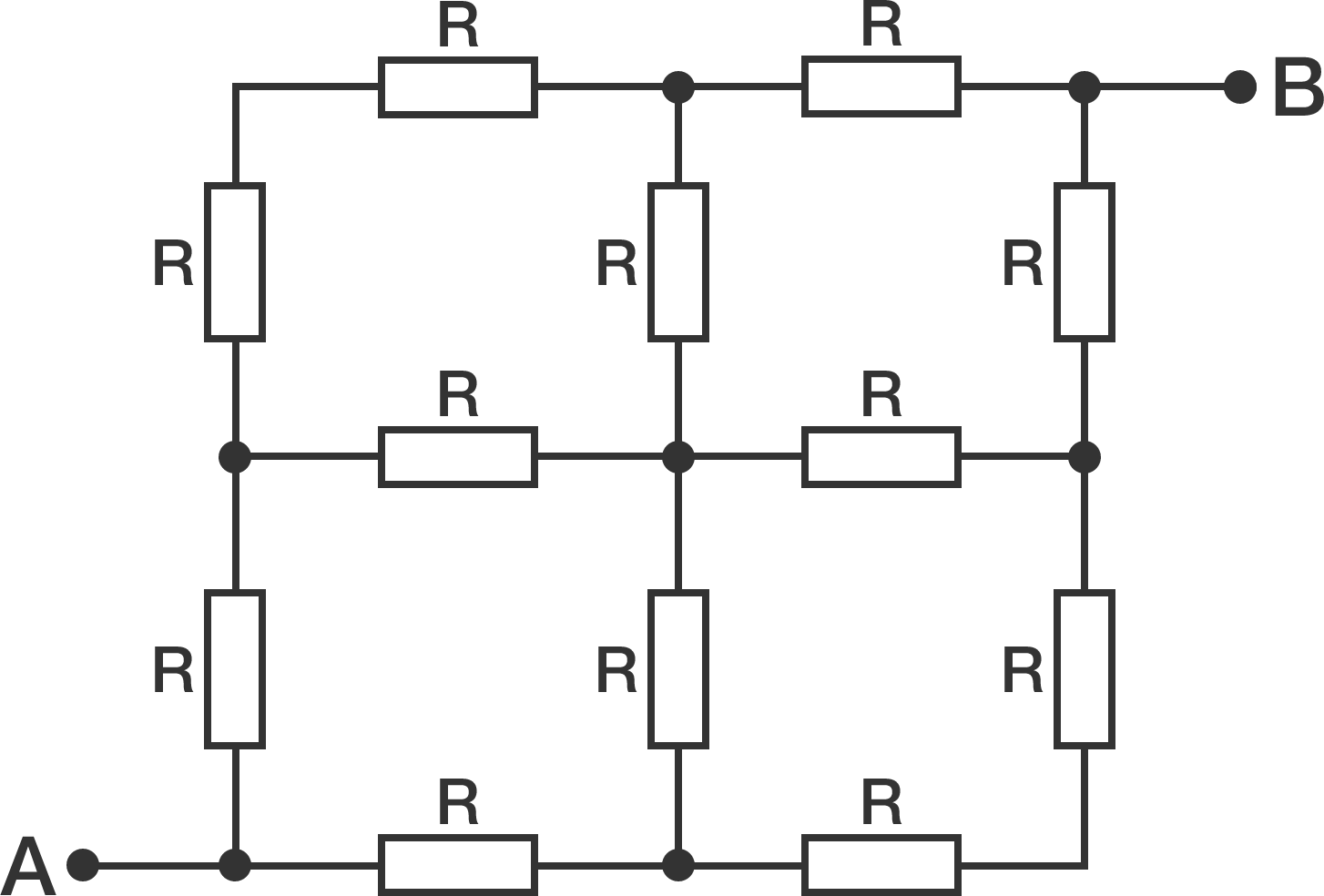# A resistor swarmAll the resistors in the above circuit have equal resistance $R = \SI[per-mode=symbol]{10}{\ohm}$. Calculate the equivalent resistance $R_e$ between points $A$ and $B$ in ohms. Submit your answer to the nearest integer.

×